# The Artist's Husband: The de Jong Attractor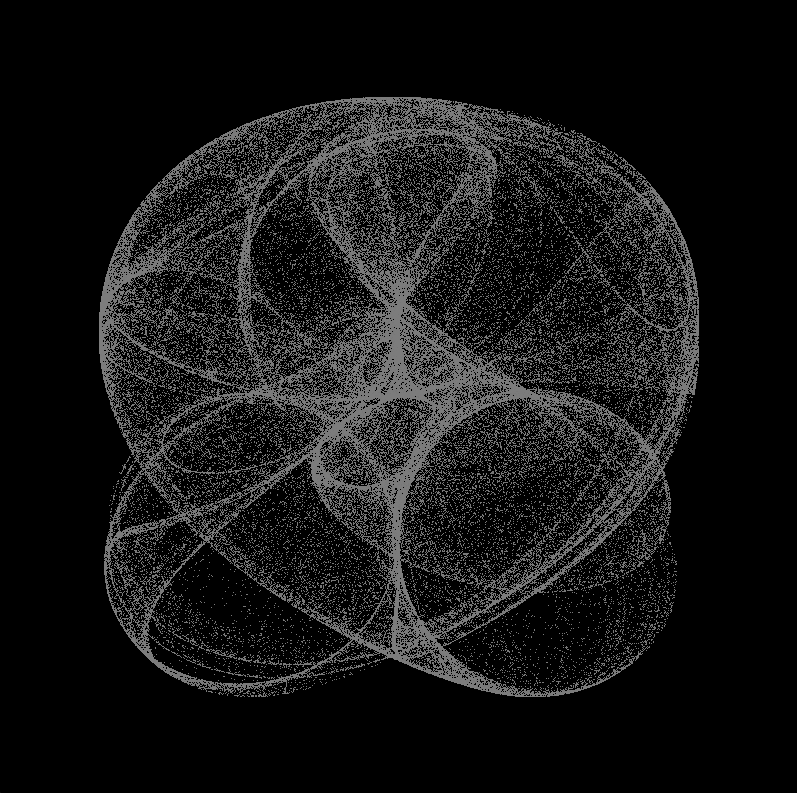In my last post , we played The Chaos Game and ended up with a Sierpinski Triangle. It’s quite nice as far as it goes, but there is not a lot of variation and visual interest beyond the initial surpise of finding it buried in the chaos at all. This time around, lets look at the de Jong attractor.

First, some terminology! An Attractor is a dynamic system with a set of numeric values to which the system tends to evolve over time, no matter what state it starts in. An attractor is called a Strange Attractor if it contains a fractal element. The Sierpinski Triangle we came up with last week is an example of a strange attractor. It doesn’t matter what your starting point is (it could be miles away from the triangle), you will eventually get pretty much the same result for any given triangle. The de Jong attractor is another example of a strange attractor.

The de Jong attractor works by starting with a random point, and modifying that point successively according to a specific set of equations. The constants a, b, c, and d are used to transform the point. Each set of values for a, b, c, and d will generate a different image.

$$x_{t+1} = sin(a * y_t) - cos(b * x_t)$$ $$y_{t+1} = sin(c * x_t) - cos(d * y_t)$$

My initial stab at this was to take my Sirepinski Triangle program from last week and make a few changes. It was straightforward, since the process is pretty much the same; we just need to change the code that transforms a point to the next point in the sequence. Here is the code:

(ns my_clj.dejong
(:require [quil.core :as q]))

(def wd 800)
(def ht 800)

(def a 1.4)
(def b -2.3)
(def c 2.4)
(def d -2.1)

(defn transform [[x y]]
[(- (q/sin (* a y)) (q/cos (* b x)))
(- (q/sin (* c x)) (q/cos (* d y)))])

(def generator
(let [x (rand)
y (rand)]
(iterate transform [x y])))

(defn setup []
(q/smooth)
(q/background 240)
(q/stroke 100 0 0)
(q/with-translation [(/ wd 2) (/ ht 2)]
(let [points 500000
scale 150]
(doseq [[x y] (take points generator)]
(q/point (* scale x) (* scale y))))))

(defn -main [& args]
(q/defsketch dejong
:title "de Jong"
:setup setup
:size [wd ht]
:features [:keep-on-top
:exit-on-close]))


This program has the four constants hardcoded, so it only generates one image: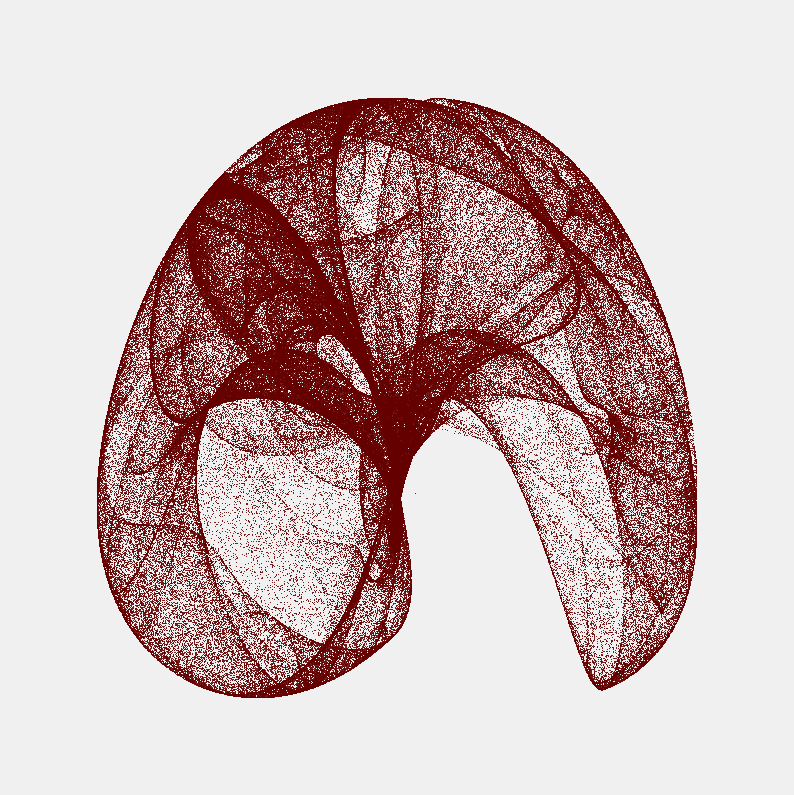Part of my goal here is to learn Clojure, so I set about modifying that program to accept the constants on the command line. Here’s what I came up with:

(ns my_clj.dejong
(:require [quil.core :as q]))

(def wd 800)
(def ht 800)

(defn rand-dj-param []
(- 3 (rand 6)))

(defn parse-argument [args pos]
(if (<= (count args) pos)
(rand-dj-param)
(if (nth args pos)
(Float/parseFloat (nth args pos))
(rand-dj-param))))

(defn process-args [args]
(map #(parse-argument args %) [0 1 2 3]))

(defn transform-fn [a b c d]
(fn [[x y]]
[(- (q/sin (* a y)) (q/cos (* b x)))
(- (q/sin (* c x)) (q/cos (* d y)))]))

(defn setup [args]
(println "a =" (nth args 0))
(println "b =" (nth args 1))
(println "c =" (nth args 2))
(println "d =" (nth args 3))
(q/smooth)
(q/background 0)
(q/stroke 0 0 200)
(q/with-translation [(/ wd 2) (/ ht 2)]
(let [points 200000
scale 150
generator (let [x (rand)
y (rand)
transform (apply transform-fn args)]
(iterate transform [x y]))]
(doseq [[x y] (take points generator)]
(q/point (* scale x) (* scale y))))))

(defn -main [& args]
(q/defsketch dejong
:title "de Jong"
:setup (fn [] (setup (process-args args)))
:size [wd ht]
:features [:keep-on-top
:exit-on-close]))


There are a few other changes here as well. The most obvious is that the setup function prints the values of the four constants that were used on the console. There’s a lot of code here devoted to finding the values for the constants on the command line, converting them from strings to numbers, and substituting random values if any are missing. Disclaimer: this is almost certainly not the best way to do this in Clojure! Just the first stab by a Clojure newbie at figuring out a way to do it!

One major difference is the function that transforms one point into the next point. The transform-fn function looks like it does that, but don’t be fooled! transform-fn accepts a vector containing the four constants, and returns another function which will accept a point and transform it to the next point in the sequence. This function is called only once, and the function it returns is what does all the work. We do this to avoid having to pass in the constants each time, making the code a bit easier to read and understand. This is common in functional programming languages like Clojure.

You run the program with an optional set of constants passed in on the command line. Four are needed, and if any are missing, random values between -3 and 3 are substituted. The image at the top of this post was generated with this command:

$lein run -m my_clj.dejong 2.5062619173168654 -2.152745266073052 2.221169409104345 -1.7962591544638  Where did I get those numbers? I ran it several times passing in no numbers, and it chose random constants. This was one of the more interesting-looking results. When you run the program, it opens a window to display the image, and it alsi displays the constants on the console so you can make a note of them if you like what you see. For example: $  lein run -m my_clj.dejong
a = 0.2586573036971309
b = 2.742585784673432
c = -2.037825586955444
d = 0.9734737216851559


…which happened to produce this: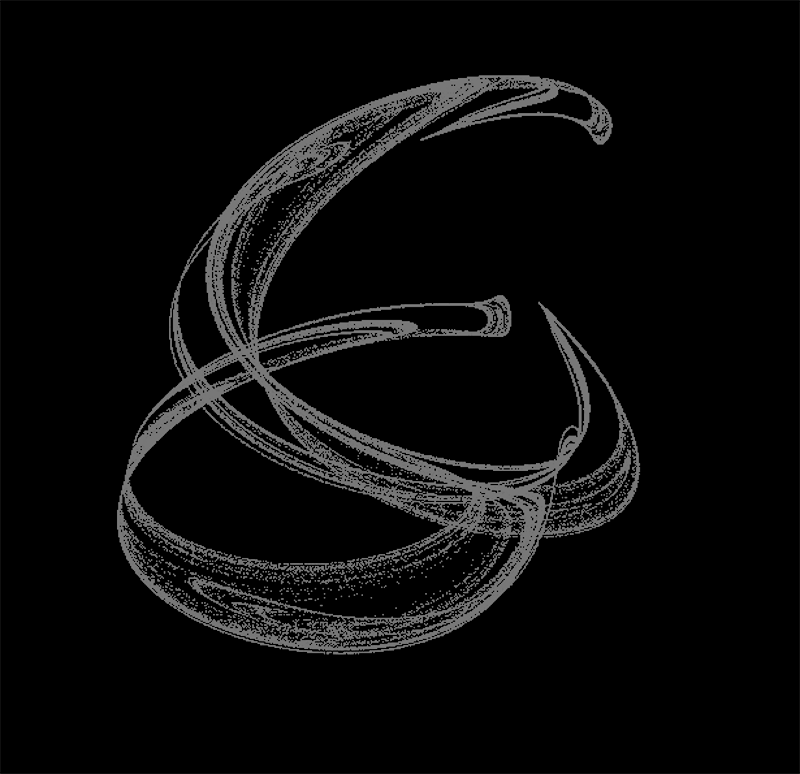When you generate these with random constants, there are two things to be aware of. First, you are going to get a lot of uninteresting results. Keep trying until you see something you like. Second, you may well be the first human ever to see a particular random image! With four 32-bit parameters, the odds of choosing any particular one is 2^128, or 1 in 340282366920938463463374607431768211456.

Here are a few more I found that I liked.

$lein run -m my_clj.dejong 2.6794055837885518 2.452123062968634 -1.7515416509942803 0.5026476820226775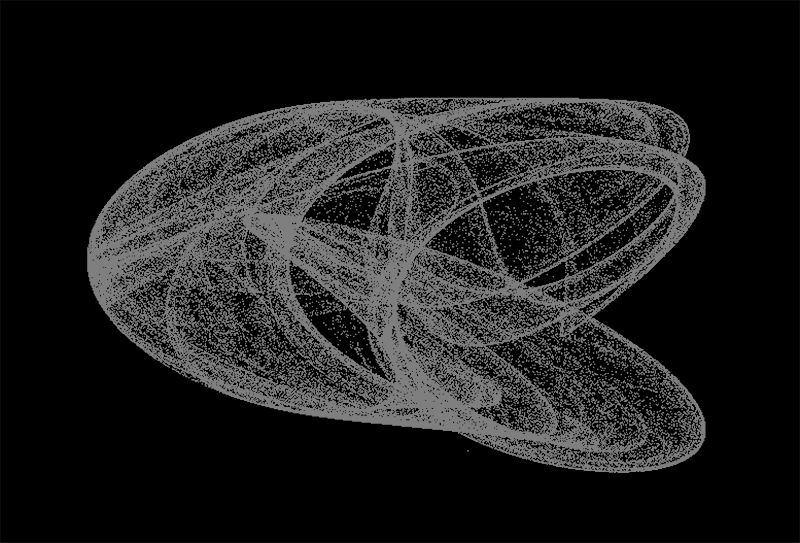$  lein run -m my_clj.dejong -2 -2 -2 -2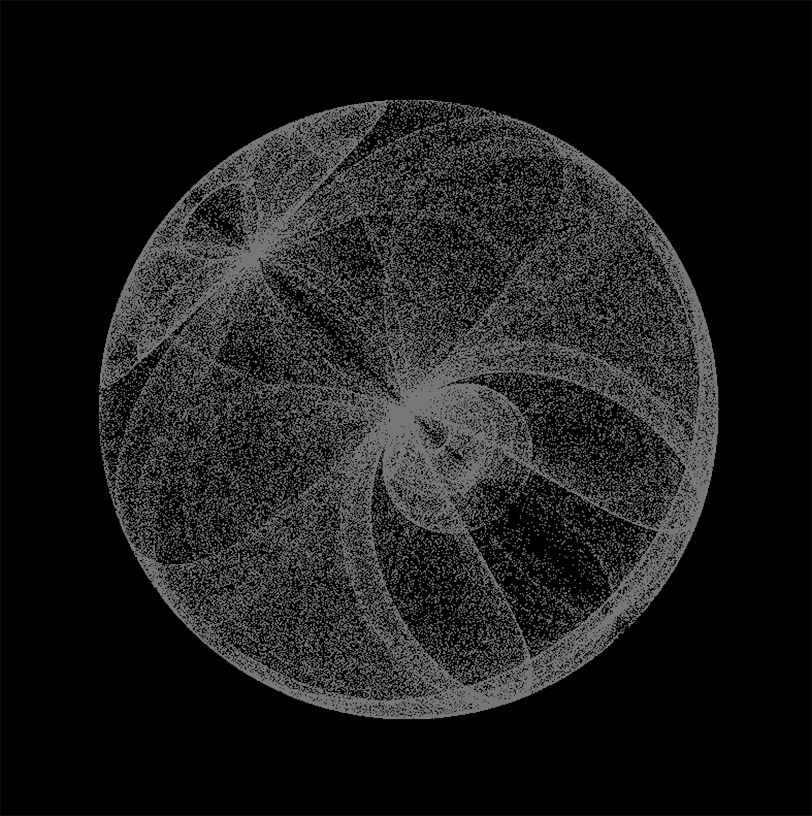lein run -m my_clj.dejong -2 -2 -1.2 2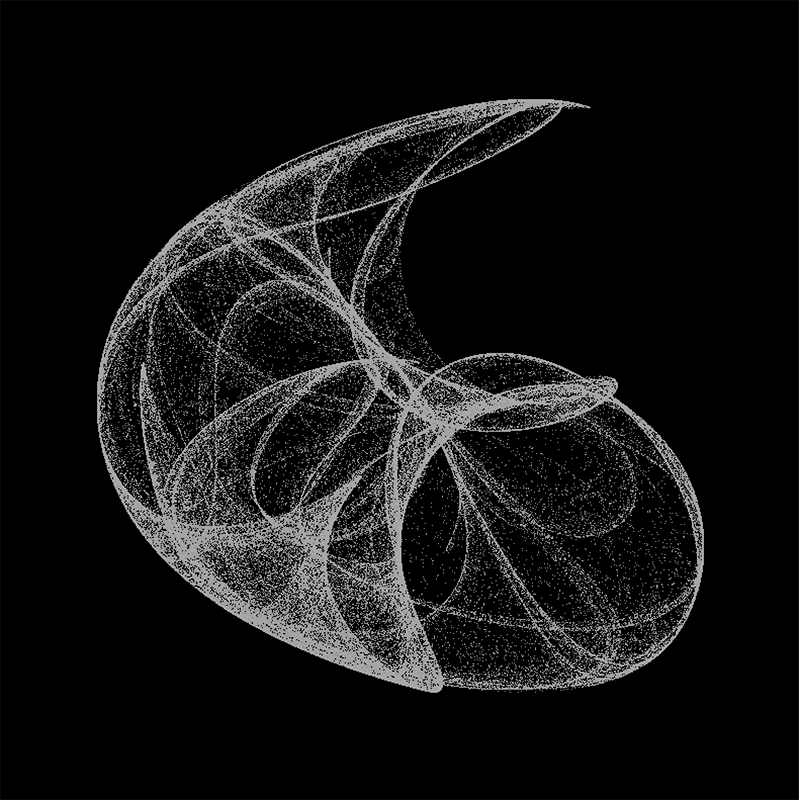Next time, I’ll explore ways to color these images better. See you then!